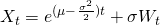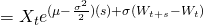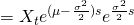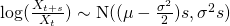# Geometric Brownian MotionThis is really important for anyone interested in Finance Modelling. As what the movie Wolf on Wall Street says:

They are referring to a geometric brownian motion.

Firstly, we will begin with the definitions.

We say that a random process,, is a geometric Brownian motion (GBM) if for allwhereis a Standard Brownian Motion
Hereis the drift andis the volatility. We writeAlso note that; This is a common technique for solving expectations.. This is very useful for simulating security prices.

Consider; Notice this expansion is similar to before.This result tells us that the expected growth rate ofis.

From the definitions of Brownian Motion introduced earlier, we extend them to Geometric Brownian motion.
1. Fix. Thenare mutually independent.
2. Paths ofare continuous as function of, meaning they do not jump.
3. For,So now lets try to do some modelling of stock prices as a geometric brownian motion.

Suppose. Clearly
1.for anyThis tells us that the limited liability of stock price is not violated.
2. The distribution ofonly depends on s and not on \$latex X_t.
We will look at the Black-Scholes option formula next time and will come back to review the geometric brownian motion for the underlying model.

Not readable? Change text.